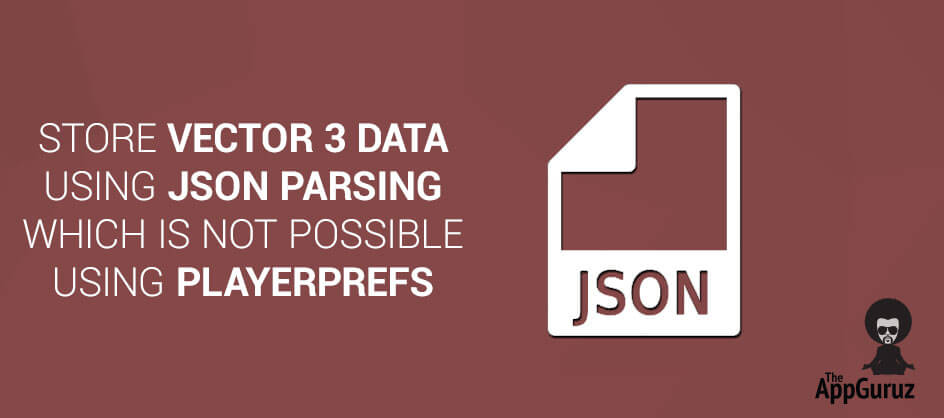## Objective

The Main Objective of this blog post is to describe how to store Vector3 data of a gameobject in Unity which cannot be stored using PlayerPrefs.

## Step 1 Understanding About PlayerPrefs

Stores and accesses player preferences between game sessions. PlayerPrefs are used to store various data in game over a session and it stores data in Integer, Float, String using its different static method

These methods are as follows:

 static void SetInt(string key, int value) Sets the value of the preference identified by key. static void SetFloat(string key, float value) Sets the value of the preference identified by key. static void SetString(string key, string value) Sets the value of the preference identified by key.

## Step 2 Understand About Vector3 Data

Used to store a Vector3 data we can use Json Parsing. There is reader and writer method of the Json Parsing to read and write the data.

• JsonFx.Json.JsonWriter.Serialize(classObject);

### Note

JsonFx.dll File is required to use Json parsing.

## Step 3 Json Parsing Implementation

Following Code Explains how to use Json Parsing:

``````using UnityEngine;
using System.Collections;
using System.Collections.Generic;

public class DataManager : MonoBehaviour
{

//sets the different kind of data into the player prefs...

//sets the coins data(position and rotation)...
public void setCoinsData(GameObject coins)
{
int coinCounter = 0;
for (int i = 0; i < coins.transform.childCount; i++)
{
coinCounter++;
string key = coins.name + "" + coinCounter;
//Coins Tag,Position,Rotation are passed in the Constructor.
CoinsData coinData = new CoinsData(key, coins.transform.GetChild(i).position, coins.transform.GetChild(i).rotation);
//String data stores data
string data = JsonFx.Json.JsonWriter.Serialize(coinData);
// Finally storing my Data i.e parsed from jason parser in Player Prefs
PlayerPrefs.SetString(coins.tag + "" + coinCounter, data);

}
}

//gets the activated gold coins data(position and rotation)...
public GameObject getCoinsData()
{
GameObject coins = GameObject.FindGameObjectWithTag("Coin");
for (int i = 0; i < coins.transform.childCount; i++) { if (coinCounter > 0)
{
string data = PlayerPrefs.GetString(coins.tag + "" + coinCounter);
// Storing Vector3 Data in Jason.
string key = coins.name + "" + coinCounter;
coinData.coinPositionData = coinData.data [key];
// Get Coin data.
coins.transform.GetChild(i).position = new Vector3(coinData.coinPositionData.position.x, coinData.coinPositionData.position.y, coinData.coinPositionData.position.z);
coins.transform.GetChild(i).rotation = new Quaternion(coinData.coinPositionData.rotation.x, coinData.coinPositionData.rotation.y, coinData.coinPositionData.rotation.z, coinData.coinPositionData.rotation.w);
coins.transform.GetChild(i).gameObject.SetActive(true);
coinCounter--;
} else
{
coins.transform.GetChild(i).gameObject.SetActive(false);
}
}
return coins;
}
class CoinsData
{
public PositionData coinPositionData = new PositionData();
// Storing Coin Data in Dictonary.
public Dictionary data = new Dictionary();
public CoinsData(string key, Vector3 coinPosition, Quaternion coinRotation)
{
// Get popstion of coin at run time
coinPositionData.position = new Cords(coinPosition.x, coinPosition.y, coinPosition.z);
coinPositionData.rotation = new Rotation(coinRotation.x, coinRotation.y, coinRotation.z, coinRotation.w);
if (key != null)
}
// Default Constructer is needed when u r using Jason Parsing.
public CoinsData()
{
}
}
//Get Coins Postion
class PositionData
{
public Cords position;
public Rotation rotation;
}
// Get Coins Cords
class Cords
{
public float x, y, z;
public Cords(float x, float y, float z)
{
this.x = x;
this.y = y;
this.z = z;
}
public Cords()
{
}
}
// Get Coin Rotation
class Rotation
{
public float x, y, z, w;
public Rotation(float x, float y, float z, float w)
{
this.x = x;
this.y = y;
this.z = z;
this.w = w;
}
public Rotation()
{
}
}
}``````

If you have got any query related to How to store a Vector3 data easily using Json Parsing which is not possible using PlayerPrefs then please comment them below we will try to solve them out.

Got an Idea of Game Development? What are you still waiting for? Contact us now and see the Idea live soon. Our company has been named as one of the Best Game Development Company in India.I am professional game developer, developing games in Unity 3D and I am very passionate about my work.